# Solution assignment 02 Fractional functions and graphs

### Assignment 2

Given the function: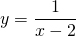Find:
the vertical asymptote;
the horizontal asymptote;
the intersection point with the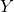-as if it exists;
the intersection point with the-as if it exists.

Based on these results sketch the result in the figure.

### Solution

This is still a quite simple fractional function. The vertical asymptote is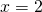. We find the horizontal asymptote by investigating the line to which the graph approaches ifand that is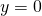. The graph intersects the-axis at. The graph has no intersection point with the-axis, because the-axis is an asymptote. This result can also be obtained by solving the equation with: this equation has no solution.
The graph is depicted in the following figure.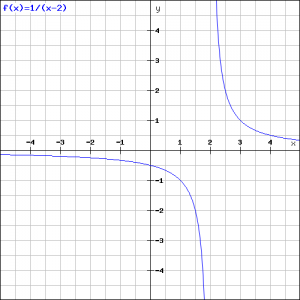0